# How to determine the number of weeks in a specific quarter

In this tutorial, we will learn three Excel functions that we need to use to achieve the goal. These three functions are listed below:

• DATE function
• EOMONTH function
• NETWORKDAY.INTL function.

## DATE Function

= DATE(year,month,day)

This function lets you combine three separate values to form a date. For example, the date January 1, 2022 would be written in this function as:

=DATE(2022,1,1)

## EOMONTH Function

=EOMONTH(start_date,months)

This function requires two arguments: start_date and months. Excel should identify the value to be input in the argument start_date as a date. So, it should only be entered as a reference to a cell that contains a recognizable date or be entered using the DATE function. The argument months means the number of months before or after the month of start_date. It could be a negative or positive value which yields a past date and future date, respectively.

This function will result to the last day of the month that the indicated number of months falls to before or after the month of the start date. For example, we want to learn the last day of the quarter starting on January 1, 2022. January is the month of the start date so the count will start on February. Therefore, we will use 2 as the argument month to get March 31, 2022. The function should be as written as below:

=EOMONTH(DATE(2022,1,1),2)

## NETWORKDAYS.INTL Function

= NETWORKDAYS.INTL(start_date,end_date,[weekend],[holidays])

The last function that we need to learn is the NETWORKDAYS.INTL function. This function returns the number of workdays between two dates using parameters to indicate which are the workdays. The days specified as weekend or holiday will not be considered as workdays and therefore will not be included in the count.

This function contains four arguments. The argument start_date is the date when the count of the number of workdays will start, while the end_date is the date when the count will end. Like in the previous function, the argument start_date and end_date should be recognizable by Excel as a date. The next argument [weekend] is an optional argument. It indicates which should be included in the number of workdays. It could be entered as the preemptive selection in Excel or as string values of seven characters that we will be discussing later. And the last argument is [holiday] which is also an optional argument. This argument is for date/s considered as special holidays that should be input manually. It should also be recognized by Excel as a date value.

For example, we want to determine the number of workdays between December 20, 2021 and December 31, 2021, then December 23, 2021 was announced as a non-working holiday. The formula should be written as:

=NETWORKDAYS.INTL(DATE(2021,12,20),DATE(2021,12,31),1,DATE(2022,12,23))

This should result to 8, which means that there are 8 working days between the given dates less the weekend, regular holidays and specially made holidays.

However, in our formula, we will not be using this function to count all the workdays in a quarter. But instead, we will use the [weekend] argument to specify one workday within a week so Excel is only counting one day per week. Therefore, the final count of the formula will actually give us the count of weeks during the quarter. To do this, we will input the argument using the string values with seven characters that I mentioned above. Each of the seven characters represent the days of the week. The first character represents Monday, the second character represents Tuesday and so on. This string can only contain characters “1” and “0”. “1” means a non-workday while “0” means a workday. Because Excel is only counting the workdays, we will designate only one “0” in our string. And remember that it should be entered in Excel with quotation marks. See the table below on how the string should look like using the different days of the week:

Now that we are knowledgeable about the three functions, let us combine these and create the formula we need to determine the number of weeks in a quarter. For our  example, we will be using the situation where we want to learn how many workweeks there are in the first quarter of 2022. To get this, we will use NETWORKDAYS.INTL function as our main function in our formula.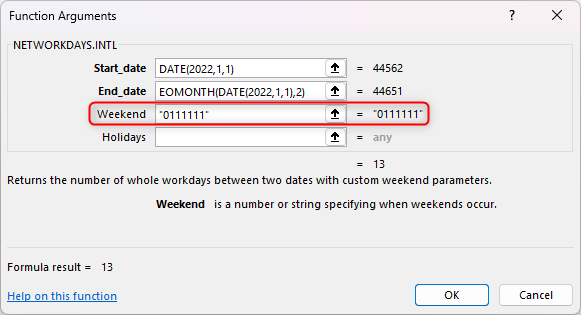If you are a project manager and you use Excel to keep track of the project timeline and schedules then you must have come across the situation when you need to calculate the number of working weeks between a quarter of the year. Excel doesn’t have a straightforward formula for this; however, we can use a combination of some functions to calculate this. It lets you save your time and trouble in counting them manually.

### Step 1 – Insert the main function we will be using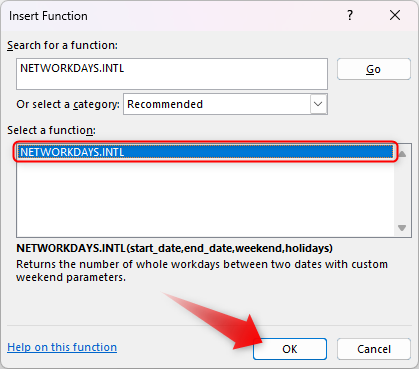– Click on the ‘Insert Function’ or the fx symbol located on the left side of the formula bar.

– Search NETWORKDAYS.INTL then click “OK”

### Step 2 – Determine the argument start_date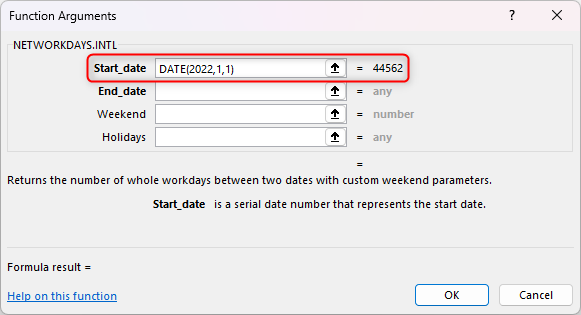– We will manually enter the start_date using the DATE function The first quarter of 2022 starts at January 1, 2022, so the first argument using the DATE function should be as written below:
DATE(2022,1,1)

### Step 3 – Determine the argument end_date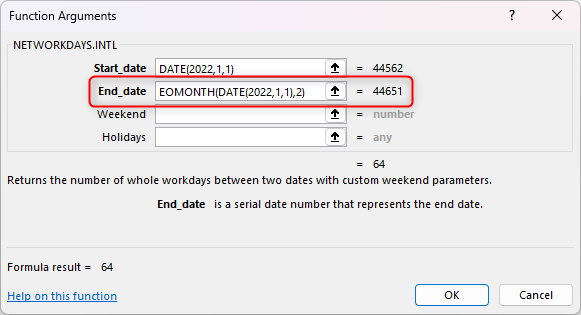– For the argument end_date we will be using the EOMONTH function. We will also be using the start_date we got for our main function.

– There are three months in a quarter. However, the months argument will start to count after the month of start_date. Since the month of our start_date is January, then the count will start in February. And we want to get the last day of March, so we will indicate this argument as 2.

– Our argument should be written as below:
EOMONTH(DATE(2022,1,1),2)

### Step 4 – Determine the [weekend]– We will be using Monday for this example, but you can use any day at your discretion. Just make sure that the string contains only one “0” and the rest are “1”. Monday string value should be as written below:
“0111111”

### Step 5 – Click OK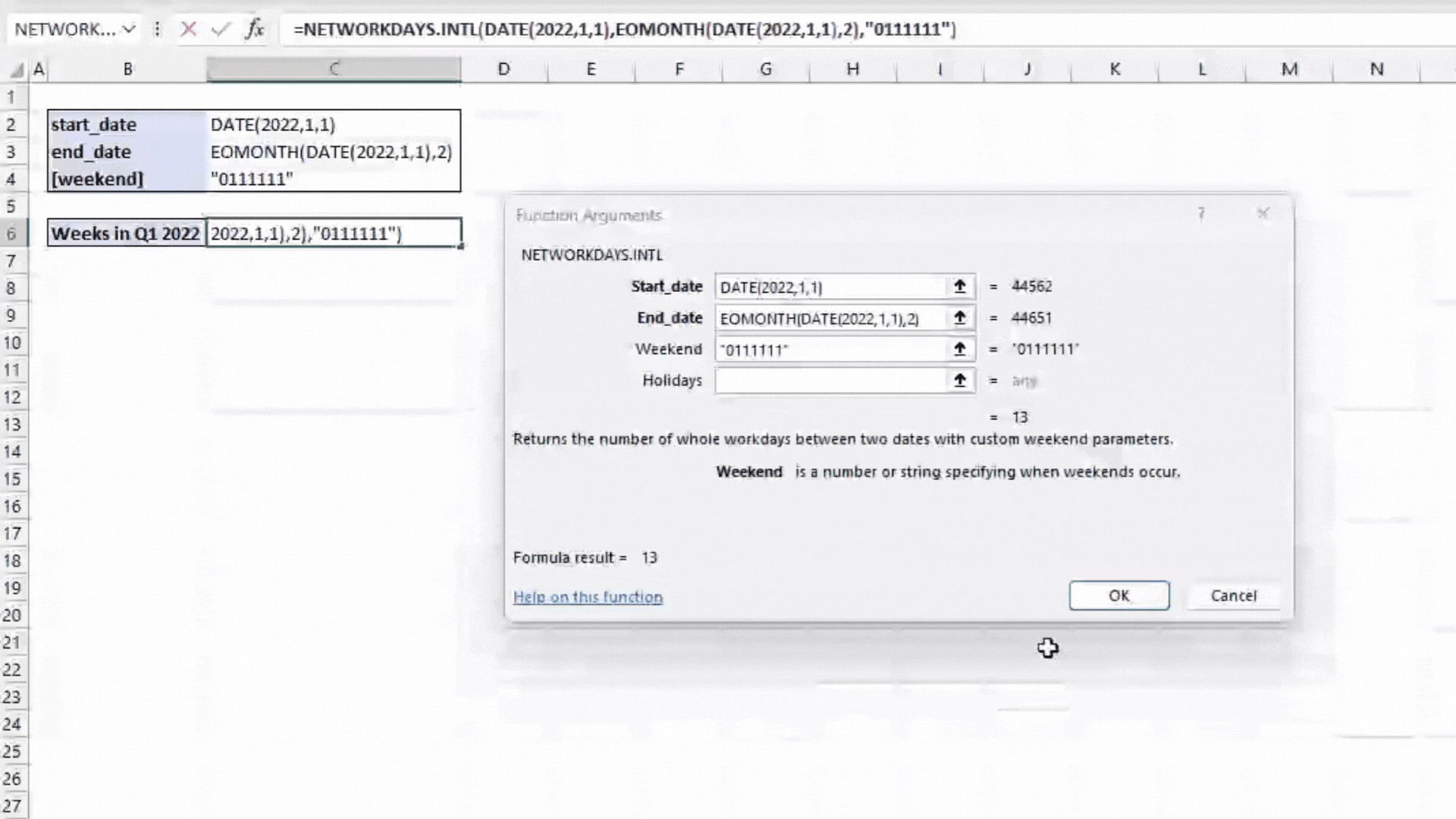– We will leave holidays blank assuming there are no special holidays.

– Click OK and it will give us a result of 13.